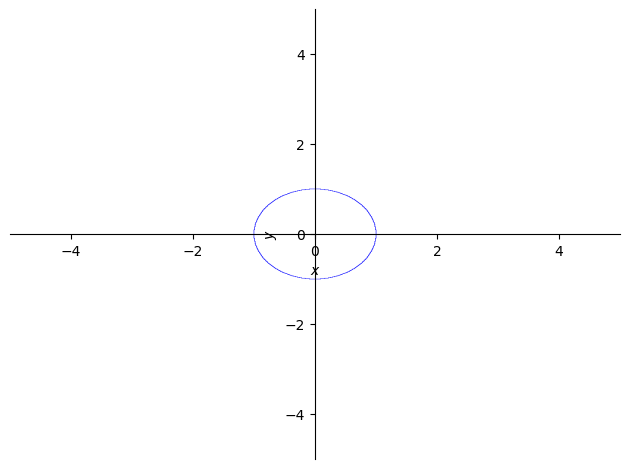# How to graph curves that are not functions (in Python, using SymPy)

See all solutions.

Assume we have an equation in which $y$ cannot be isolated as a function of $x$. (The standard example is the formula for the unit circle, $x^2+y^2=1$.) We would still like to be able to use software to plot such curves. How?

## Solution

This answer assumes you have imported SymPy as follows.

1
2
from sympy import *                   # load all math functions
init_printing( use_latex='mathjax' )  # use pretty math output


Let’s consider the example of the unit circle, $x^2+y^2=1$.

To plot it, SymPy first expects us to move everything to the left-hand side of the equation, so in this case, we would have $x^2+y^2-1=0$.

We then use that left hand side to represent the equation as a single formula, and we can plot it with SymPy’s plot_implicit function.

1
2
3
var( 'x y' )
formula = x**2 + y**2 - 1   # to represent x^2+y^2=1
plot_implicit( formula )1
<sympy.plotting.plot.Plot at 0x7f67130cb460>